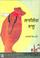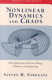### Differential Equations and Stability Theory

#### Course Description

Linear systems of differential equations. Equilibrium points. Classification of phase portraits. Stability . Nonlinear systems. Periodic solutions and limit cycles. Energy method. Ljapunov stability and instability theorems. Robustness of systems. Bifurcations. Types and classification of partial differential equations of the second order. Heat conduction equation. Wave equation. Laplace equation.

#### General Competencies

This is an introduction to the qualitative theory of differential equations. The subject gives a mathematical background to the nonlinear control. This subject is also an introduction to partial differential equations of the second order.

#### Learning Outcomes

1. Define fundamental notions related to qualitative theory of ordinary differential equations and fundamental notions related to partial differential equations of second order.
2. Relate knowledge achived in linear algebra to behaviour of linear and nonlinear systems
3. Compare knowledge from other courses with mathematical knowledge about differential equations.
4. Recognize difference between analytic, numeric and qualitative approach to differential equations.
5. Analyze a problem, he/she will be able to modify the methods and apply to them
6. Estimate is his/her solution in accordance to theoretical basis

#### Forms of Teaching

Lectures

Lectures.

Exams

Midexam, exam, seminar

Consultations

Consulting.

Seminars

seminar.

By decision of the Faculty Council, in the academic year 2019/2020. the midterm exams are cancelled and the points assigned to that component are transferred to the final exam, unless the teachers have reassigned the points and the grading components differently. See the news for each course for information on knowledge rating.

Continuous Assessment Exam
Homeworks 0 % 10 % 0 % 0 %
Class participation 0 % 4 % 0 % 0 %
Seminar/Project 0 % 6 % 0 % 0 %
Mid Term Exam: Written 0 % 35 % 0 %
Final Exam: Written 0 % 45 %
Exam: Written 0 % 100 %

#### Week by Week Schedule

1. Eigenvalues and the Jordan form of a matrix. Linear systems of differential equations in the plane.
2. Klasifikacija faznih portreta linearnih sustava oko Classification of phase portraits of linear systems near the equilibrium points. Stability and asymptotical stability.
3. Closed trajectory and limit cycle. Linearization, Hartman-Grobman theorem.
4. Stability theory. Invariant manifolds.
5. Bendixson theorem, Duffing equation. Poincare index theory
6. Dissipative and Conservative systems, energy method. Liouville theorem.
7. Lyapunov stability and instability theorems. Poincare Bendixson theorem.
8. Exam
9. Blowing-up method. Robustness of the system. Normal form.
10. Bifurcation. Hopf-Takens bifurcation. Examples of higher-dimensional systems.
11. Types of partial differential equations. Classification of partial differential equations of the second order. Heat conduction equation. Nonstacionary heat conduction. Fouriers method.
12. Method of decomposition by eigenfunctions. Application of Laplace transform to the equation of parabolic type.
13. Wave equation. Equation of oscillation of a string. D´ Alambert formula. Application of Fouriers method of separation of variables to the wave equation.
14. Laplacian in Cartesian, cylindrical and spherical coordinates. Laplace equation. Boundary-value problems for Laplace equation. Dirichlet problem for disc and ring.
15. Final exam

#### Study Programmes

Computer Engineering (profile)
Mathematics and Science (2. semester)
Computer Science (profile)
Mathematics and Science (2. semester)
Control Engineering and Automation (profile)
Mathematics and Science (2. semester)
Electrical Engineering Systems and Technologies (profile)
Mathematics and Science (2. semester)
Electrical Power Engineering (profile)
Mathematics and Science (2. semester)
Electronic and Computer Engineering (profile)
Mathematics and Science (2. semester)
Electronics (profile)
Mathematics and Science (2. semester)
Information Processing (profile)
Mathematics and Science (2. semester)
Software Engineering and Information Systems (profile)
Mathematics and Science (2. semester)
Telecommunication and Informatics (profile)
Mathematics and Science (2. semester)
Wireless Technologies (profile)
Mathematics and Science (2. semester)

#### LiteratureLawrence Perko (2001.), Differential Equations and Dynamical Systems, SpringerSteven H. Strogatz (2000.), Nonlinear Dynamics and Chaos, With Applications to Physics, Biology, Chemistry, and Engineering, Perseus Books PublishihgStanley J. Farlow (1982.), Partial Differential Equations for Scientists and Engineers Stanley J. Farlow John Wiley & Sons 1982, Stanley J. Farlow John Wiley & SonsLuka Korkut, Vesna Županović (2009.), Diferencijalne jednadžbe i teorija stabilnosti, ElementR. Kent Nagle, Edward B. Saff (1996.), Fundamentals of Differential Equations and Boundary Value Problems, Addison-Wesley Publishing Company

#### General

ID 34555
Summer semester
4 ECTS
L0 English Level
L1 e-Learning
45 Lectures
0 Exercises
0 Laboratory exercises
0 Project laboratory